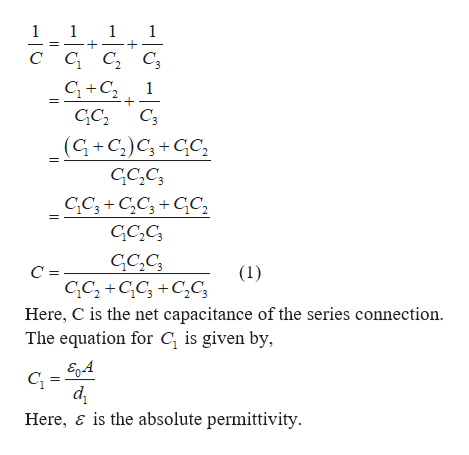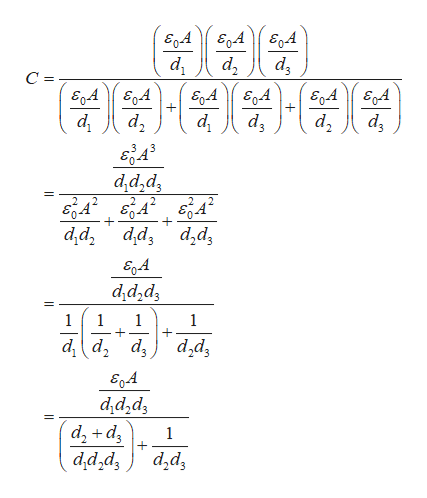# Three parallel-plate capacitors are constructed, each havingthe same plate area A and with C1 having plate spacing d1,C2 having plate spacing d2, and C3 having plate spacing d3.Show that the total capacitance C of the three capacitors connectedin series is the same as a capacitor of plate area A andwith plate spacing d = d1 +d2 x d3.

Question
1 views

Three parallel-plate capacitors are constructed, each having
the same plate area A and with C1 having plate spacing d1,
C2 having plate spacing d2, and C3 having plate spacing d3.
Show that the total capacitance C of the three capacitors connected
in series is the same as a capacitor of plate area A and
with plate spacing d = d1 +d2 x d3.

check_circle

Step 1

The equation for the net capacitance of the series connection is given by,help_outlineImage TranscriptioncloseC C C, C3 C +C, +. GC, C3 (G +C;)C; + GC, GC,C; CC; +C,C; + GC, GC,C; GC,C; (1) GC, +GC; +C,C3 Here, C is the net capacitance of the series connection. The equation for C, is given by, d Here, ɛ is the absolute permittivity. || fullscreen
Step 2

The equation for C2 is given by,

Step 3

Putting the above three equat...help_outlineImage TranscriptioncloseE,A d , d, d, =- E,A E,4 аалЕ E,A E,A d, dz d, d, d,d,dz вд е4 в4 d,d, d,d, d,d, E,A d;d,d3 d d, d, d,d, 2. d,d,dz d, +d, d,d,dz d,dz fullscreen

### Want to see the full answer?

See Solution

#### Want to see this answer and more?

Solutions are written by subject experts who are available 24/7. Questions are typically answered within 1 hour.*

See Solution
*Response times may vary by subject and question.
Tagged in

### Electrostatic Potential and Capacitance